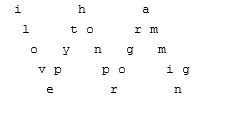# Program to convert a string to zigzag string of line count k in python

Suppose we have a string s and another value k, We have to find a new string by taking each character from s and starting diagonally from top left to bottom right until reaching the kth line, then go up to top right, and so on.

So, if the input is like s = "ilovepythonprogramming" k = 5, then the output will beTo solve this, we will follow these steps:

• line := a new map
• cnt := 0
• delta := 1
• for each index i and character c in s, do
• insert (c, i) at the end of line[cnt]
• cnt := cnt + delta
• if cnt is same as k, then
• delta := -1
• cnt := k - 2
• if cnt is same as 0, then
• delta := 1
• ans := a new list
• for each key i and value c in line, do
• prefix := a list of size same as s and fill that with single blank spaces
• for each pair (x, y) in c, do
• prefix[y] := x
• join each element present in prefix and insert it into ans
• return a new string by adding a new line between each consecutive elements in ans

Let us see the following implementation to get better understanding:

## Example

Live Demo

from collections import defaultdict
class Solution:
def solve(self, s, k):
line = defaultdict(list)
cnt = 0
delta = 1
for i, c in enumerate(s):
line[cnt].append((c, i))
cnt += delta
if cnt == k:
delta = -1
cnt = k - 2
if cnt == 0:
delta = 1

ans = []

for i, c in line.items():
prefix = [" "] * (len(s))
for x, y in c:
prefix[y] = x
ans.append("".join(prefix))

return "\n".join(ans)

ob = Solution()
s = "ilovepythonprogramming"
k = 5
print(ob.solve(s, k))

## Input

"ilovepythonprogramming", 5

## Output